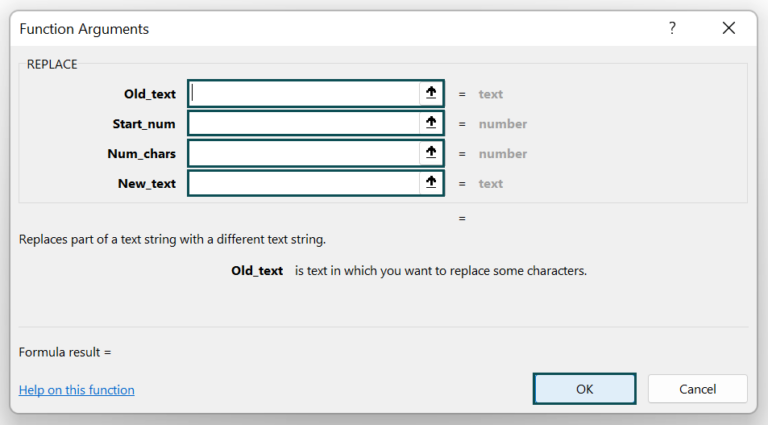# Excel REPLACE FunctionArticle byExcelMojo Team## What Is REPLACE Function In Excel?

The REPLACE function in Excel replaces a sequence of characters in a string with another set of characters. The REPLACE function is an inbuilt function that is similar to the substitute function. The REPLACE function in Excel is categorized under Excel TEXT functions.

For example, consider the below table with values in cell A2. We need to use the below steps to obtain the result using REPLACE Function.

The steps used to replace values in Excel are as follows:

• Select the cell to display the result. In this example, we have selected cell B2.
• Enter the values in cell B2. The complete formula is, =REPLACE(A2,6,6,“Methew”).
• Press Enter key.
• The output is returned as ‘John Methew’ in cell B2.

Likewise, we can replace the values using Excel REPLACE Function.

###### Key Takeaways
• Excel REPLACE Function is used to replace the text string from a given data,
• The excel REPLACE formula is =REPLACE(old_text,start_num,num_chars,new_text), where all the arguments are mandatory.
• To select the cells that we want to convert to text, we must not have more than one column.
• The “#VALUE” error occurs if the start_num argument or num_chars argument is a non-numeric or negative value.
• In the REPLACE function, the start argument cannot have a non-numeric or a negative value and the number_of_chars cannot have a non-numeric or a negative value.

### REPLACE() Excel Formula

The syntax of the Excel REPLACE formula is:

where,

• old_text: It denotes the text to be replaced
• start_num: It indicates the position, within old_text argument. It is the first character we want to replace.
• num_chars: It shows the number of characters we want to replace.
• new_text: It is the text which we want to replace in place of the selected text.

Unlike other functions, all the arguments in this function are mandatory.

### How To Use REPLACE Excel Function?

1. Access from the Excel ribbon

1. Choose the empty cell which will contain the result.
2. Go to the Formulas tab.
3. Select the Text option from the menu.
4. Select REPLACE from the drop-down menu.
5. The window called Function Arguments appears.
6. As the number of arguments, enter the value in the Old_text, Start_num, Num_chars, and new_text
7. Select OK.

2. Enter the worksheet manually

1. Select an empty cell for the output.
2. Type =REPLACE( in the selected cell.
3. Alternatively, type =R and double-click on the REPLACE function from the list of suggestions shown by Excel.
4. Enter the arguments as excel cell references or direct values.
5. Close the parenthesis and press Enter key.

Let us take an example to understand this function. The below table shows text string in A2. We need to use the below steps to replace the text using Excel REPLACE Function.

The steps to replace the strings by Excel REPLACE Function are as follows:

1. Select the cell where we will enter the formula and calculate the result. The selected cell, in this case, is cell B2.

2. Enter the excel REPLACE formula in cell B2.

The entered value in the old_text argument is cell A2.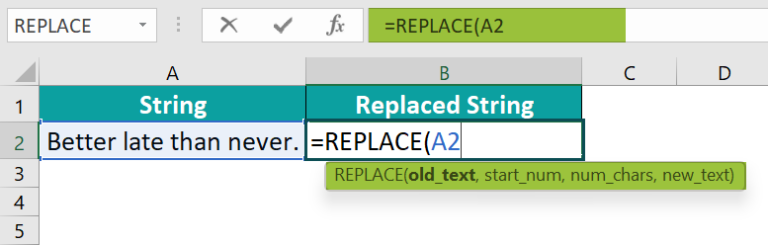3. The entered value of the start_num argument is 8.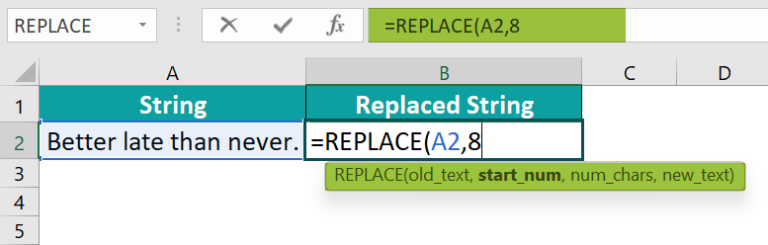4. The entered value of the num_chars argument is 4.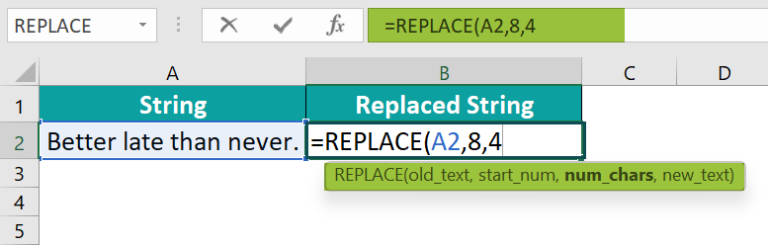5. The entered value of the new_text argument is early.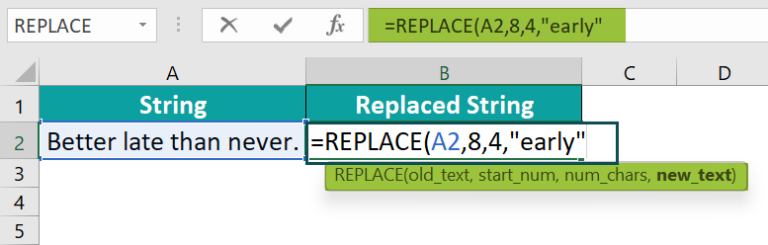6. The complete formula is =REPLACE(A2,8,4,“early”).

The function has returned the result as ‘Better early than never’ in cell B2, as shown in the following image.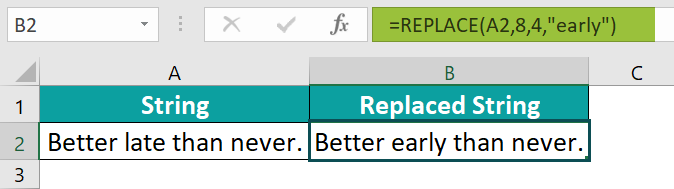Similarly, we can use Excel REPLACE Function to replace the text string.

### Examples

#### Example #1 – Replace A String

The following table shows text string in cell A2. We need to use the below steps to replace the text using Excel REPLACE Function.

The steps to replace the strings using REPLACE function are as follows:

Step 1: Select the cell where we will enter the formula and calculate the result. The selected cell, in this case, is cell B2.

Step 2: Enter the REPLACE formula in cell B2. The entered value of the old_text argument is cell A2.

Step 3: The entered value of the start_num argument is 7.

Step 4: The entered value of the num_chars argument is 3.

Step 5: The entered value of the new_text argument is Tart.

Step 6: The complete formula is =REPLACE(A2,7,3,“Tart”).

The function has returned the result in cell B2, as shown in the following image.

Likewise, we can use Excel REPLACE Function to replace text.

#### Example #2 – Replace A Substring

The below table shows text string in cell A2. We need to use the below steps to replace using Excel REPLACE Function.

The steps to replace the strings using Excel REPLACE Function are as follows:

Step 1: Select the cell where we will enter the formula and calculate the result. The selected cell, in this case, is cell B2.

Step 2: Enter the REPLACE formula in cell B2. The entered value of the old_text argument is cell A2.

Step 3: The entered value of the start_num argument is 5.

Step 4: The entered value of the num_chars argument is 6.

Step 5: The entered value of the new_text argument is Facebook.

Step 6: The entered complete formula is =REPLACE(A2,5,6,“facebook”).

The function has returned the result in cell B2, as shown in the following image.

Similarly, we can use Excel REPLACE Function to replace text.

#### Example #3 – Replace A Single Character

The below table shows text string in cell A2. We need to use the below steps to replace the text using Excel REPLACE Function.

The steps to replace the strings by the REPLACE in Excel are as follows:

Step 1: Select the cell where we will enter the formula and calculate the result. The selected cell, in this case, is cell B2.

Step 2: Enter the REPLACE formula in cell B2. The entered value of the old_text argument is cell A2.

Step 3: The entered value of the start_num argument is 5.

Step 4: The entered value of the num_chars argument is 1.

Step 5: The entered value of the new_text argument is u.

Step 6: The complete formula is =REPLACE(A2,5,1,“u”).

The function has returned the result in cell B2, as shown in the following image.

Similarly, we can use REPLACE Function to replace text.

#### Example #4 – Replace Numbers

The below table shows text string in cell A2. We need to use the below steps to replace the text using Excel REPLACE Function.

The steps to replace the strings by the REPLACE in Excel are as follows:

Step 1: Select the cell where we will enter the formula and calculate the result. The selected cell, in this case, is cell B2.

Step 2: Enter the REPLACE formula in cell B2. The entered value of the old_text argument is cell A2.

Step 3: The entered value of the start_num argument is 1.

Step 4: The entered value of the num_chars argument is 1.

Step 5: The entered value of the new_text argument is 1.

Step 6: The entered complete formula is =REPLACE(A2,1,1,“1”).

The function has returned the result in cell B2, as shown in the following image.

Similarly, we can use REPLACE Function to replace text.

#### Example #5 – Remove A String

The below table shows text string in cell A2. We need to use the below steps to remove the string using Excel REPLACE Function.

The steps to remove the string using Excel REPLACE Function are as follows:

Step 1: Select the cell where we will enter the formula and calculate the result. The selected cell, in this case, is cell B2.

Step 2: Enter the REPLACE formula in cell B2. The entered value of the old_text argument is cell A2.

Step 3: The entered value of the start_num argument is 1.

Step 4: The entered value of the num_chars argument is 14.

Step 5: The entered value of the new_text argument is “ ”.

Step 6: The complete formula is =REPLACE(A2,1,14,“ ”).

The function has removed the text string in cell B2, as shown in the following image.

Similarly, we can use Excel REPLACE Function to remove text.

### Common Errors With REPLACE Function

The below table shows text string in cell A2. We need to use the below steps to detect the Common Error with Excel REPLACE function.

The steps to detect the error using Excel REPLACE function are as follows:

Step 1: Select the cell where we will enter the formula and calculate the result. The selected cell, in this case, is cell B2.

Step 2: Enter the excel REPLACE function formula in cell B2. The entered value of the old_text argument is cell A2.

Step 3: The entered value of the start_num argument is 0.

Step 4: The entered value of the num_chars argument is 1.

Step 5: The entered value of the new_text argument is “ ”.

Step 6: The complete formula is =REPLACE(A2,0,1,“ ”).

The function has returned #VALUE! error in cell B2, as shown in the following image.

It is because, the value of start_num should be greater than 0.

Similarly, we can use Excel REPLACE Function to replace text.

1. What does the REPLACE Function do in Excel?

The Excel REPLACE function is designed to replace the text strings and returns a text string. The REPLACE function allows swapping one or several characters in a text string with another character or a set of characters.

The excel REPLACE formula is
=REPLACE(old_text,start_num,num_chars,new_text).

For example, consider the below table with a text string in cell A2. We need to apply the REPLACE function to replace the text.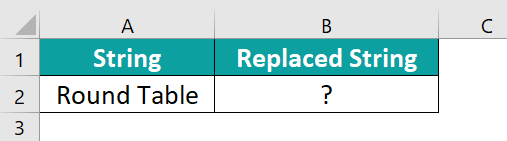The steps used to replace values in Excel are as follows:

Step 1: Select the cell to display the result. In this example, we have selected cell B2.

Step 2: Enter the values in cell B2.
The complete formula is =REPLACE(A2,1,6,“Center ”).
The output is returned as ‘Center Table’ in cell B2.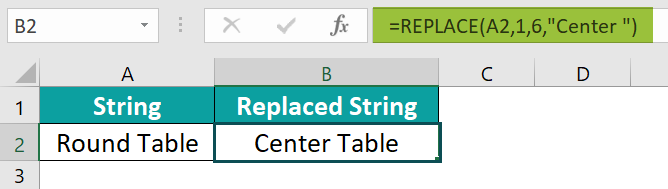Similarly, we can use Excel REPLACE Function to replace the text.

2. Why is the REPLACE Function not working in Excel?

The REPLACE function can be made to work in Excel with the following suggestions;
· Ensure the correct cell is selected.
· Unmark match entire cell content.
· Remove Filter.
· Delete unwanted spaces.
· Unprotect the sheet.
· Repair corrupted Excel sheet.

3. Where is the REPLACE function in Excel?

We can insert Excel REPLACE function using the following steps:
1. Choose the empty cell which will contain the result.
2. Go to the Formulas tab.
3. Select the Text group.
4. Select REPLACE from the drop-down menu.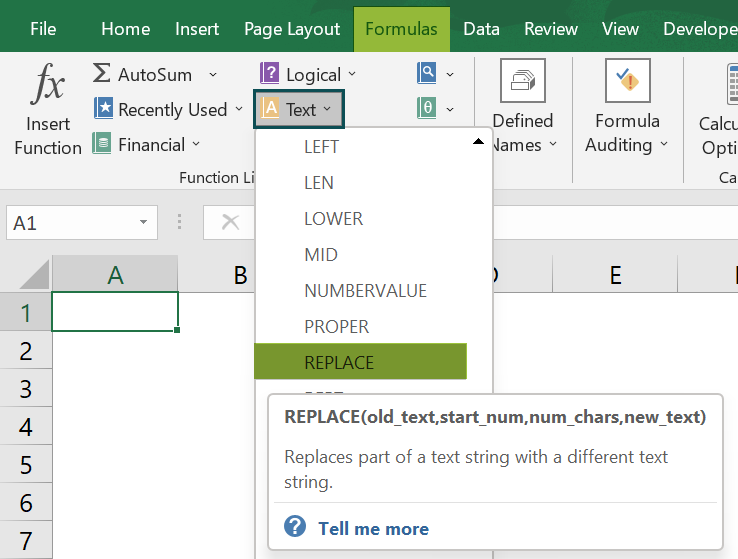5. A window called Function Arguments appears.
6. As the number of arguments, enter the value in the Old_text, Start_num, Num_chars, and New_text.
7. Select OK.